JEE  >  Test: MCQs (One or More Correct Option): The Solid State & Surface Chemistry | JEE Advanced

# Test: MCQs (One or More Correct Option): The Solid State & Surface Chemistry | JEE Advanced

Test Description

## 8 Questions MCQ Test Chemistry 35 Years JEE Main & Advanced Past year Papers | Test: MCQs (One or More Correct Option): The Solid State & Surface Chemistry | JEE Advanced

Test: MCQs (One or More Correct Option): The Solid State & Surface Chemistry | JEE Advanced for JEE 2023 is part of Chemistry 35 Years JEE Main & Advanced Past year Papers preparation. The Test: MCQs (One or More Correct Option): The Solid State & Surface Chemistry | JEE Advanced questions and answers have been prepared according to the JEE exam syllabus.The Test: MCQs (One or More Correct Option): The Solid State & Surface Chemistry | JEE Advanced MCQs are made for JEE 2023 Exam. Find important definitions, questions, notes, meanings, examples, exercises, MCQs and online tests for Test: MCQs (One or More Correct Option): The Solid State & Surface Chemistry | JEE Advanced below.
 1 Crore+ students have signed up on EduRev. Have you?
*Multiple options can be correct
Test: MCQs (One or More Correct Option): The Solid State & Surface Chemistry | JEE Advanced - Question 1

### Which of the following statement(s) is (are) correct?(1998 - 2 Marks)

Detailed Solution for Test: MCQs (One or More Correct Option): The Solid State & Surface Chemistry | JEE Advanced - Question 1

The crystals of CsCl has body - centred cubic unit cell. Hence, each ion in this structure has coordination number of eight.

NOTE : In case of crystals of NaCl two
interpenetrating face-centred cubic lattices are
present, one of these is composed entirely of Na+ ions and the other of Cl ions. Each Na+ ion is located half way between two Cl ions and viceversa. A unit cell of NaCl crystal has Cl ions at the corners as well as at the face centres and Na+ ions are located in
octahedral voids. On each edge of cubic unit cell, there are two Cl ions and one Na+ ions. Hence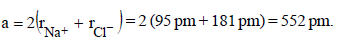*Multiple options can be correct
Test: MCQs (One or More Correct Option): The Solid State & Surface Chemistry | JEE Advanced - Question 2

### The correct statement(s) regarding defects in solids is (are)           (2009S)

Detailed Solution for Test: MCQs (One or More Correct Option): The Solid State & Surface Chemistry | JEE Advanced - Question 2

(b,c)
Frenkel defect is a dislocation effect, observed when the size of the cation and anion differ largely. F-center is created when an anion is lost from the lattice and vacancy is filled by trapping of an electron. Schottky defect changes the density of a crystalline solid.

*Multiple options can be correct
Test: MCQs (One or More Correct Option): The Solid State & Surface Chemistry | JEE Advanced - Question 3

### The correct statement(s) pertaining to the adsorption of agas on a solid surface is (are)        (2011)

Detailed Solution for Test: MCQs (One or More Correct Option): The Solid State & Surface Chemistry | JEE Advanced - Question 3

(a, b, d)
(a) ΔH is negative for adsorption
(b) Fact based
(d) Chemical bonds are stronger than vander Waal’s forces, so chemical adsorption is more exothermic

*Multiple options can be correct
Test: MCQs (One or More Correct Option): The Solid State & Surface Chemistry | JEE Advanced - Question 4

Choose the correct reason(s) for the stability of the lyophobiccolloidal particles. (2012)

*Multiple options can be correct
Test: MCQs (One or More Correct Option): The Solid State & Surface Chemistry | JEE Advanced - Question 5

The given graphs/data I, II, III and IV represent general trends observed for different physisorption and chemisorption processes under mild conditions of temperature and presure.

Which of the following choice(s) about I, II, III and IV is (are) correct ?      (2012)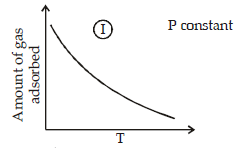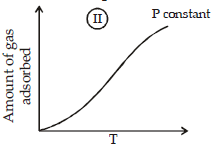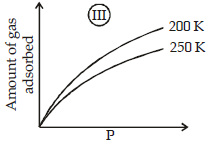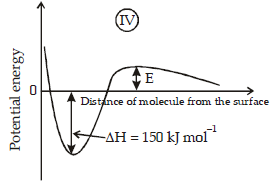Detailed Solution for Test: MCQs (One or More Correct Option): The Solid State & Surface Chemistry | JEE Advanced - Question 5

(a, c)
Graph (I) and (III) represent physisorption because, in
physisorption, the amount of adsorption decreases with the increase of temperature and increases with
the increase of pressure.
Graph (II) represent chemisorption, because in chemisorption amount of adsorption increases with the
increase of temperature. Graph (IV) is showing the formation of a chemical bond, hence chemisorption.

*Multiple options can be correct
Test: MCQs (One or More Correct Option): The Solid State & Surface Chemistry | JEE Advanced - Question 6

If the unit cell of a mineral has cubic close packed (ccp) array of oxygen atoms with m fraction of octahedral holes occupied by aluminium ions and n fraction of tetrahedral holes occupied by magnesium ions, m and n, respectively, are              (JEE Adv. 2015)

Detailed Solution for Test: MCQs (One or More Correct Option): The Solid State & Surface Chemistry | JEE Advanced - Question 6

In ccp, O2– ions are 4.
Hence total negative charge = –8
Let Al3+ ions be x, and Mg2+ ions be y.
Total positive charge = 3x + 2y
⇒ 3x + 2y = 8
This relation is satisfied only by x = 2 and y = 1.
Hence number of Al3+ = 2.
and number of Mg2+ = 1.
⇒ n = fraction of octahedral holes occupied by Al3+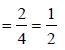and m = fraction of tetrahedral holes occupied by Mg2+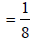*Multiple options can be correct
Test: MCQs (One or More Correct Option): The Solid State & Surface Chemistry | JEE Advanced - Question 7

When O2 is adsorbed on a metallic surface, electron transferoccurs from the metal to O2. The true statement(s) regardingthis adsorption is(are) (JEE Adv. 2015)

Detailed Solution for Test: MCQs (One or More Correct Option): The Solid State & Surface Chemistry | JEE Advanced - Question 7

Reaction on metal surface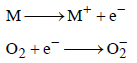This is an example of chemisorption

*Multiple options can be correct
Test: MCQs (One or More Correct Option): The Solid State & Surface Chemistry | JEE Advanced - Question 8

The CORRECT statement(s) for cubic close packed (ccp)three dimensional structure is (are) (JEE Adv. 2016)

Detailed Solution for Test: MCQs (One or More Correct Option): The Solid State & Surface Chemistry | JEE Advanced - Question 8

CCP is ABC ABC …… type packing
(A) In topmost layer, each atom is in contact with 6 atoms in same layer and 3 atoms below this layer

(B) Packing fraction =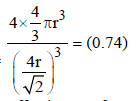(C) Each FCC unit has effective no of atoms = 4
Octahedral void = 4
Tetrahedral void = 8
(D) 4r = a√2

## Chemistry 35 Years JEE Main & Advanced Past year Papers

142 docs|66 tests
 Use Code STAYHOME200 and get INR 200 additional OFF Use Coupon Code
Information about Test: MCQs (One or More Correct Option): The Solid State & Surface Chemistry | JEE Advanced Page
In this test you can find the Exam questions for Test: MCQs (One or More Correct Option): The Solid State & Surface Chemistry | JEE Advanced solved & explained in the simplest way possible. Besides giving Questions and answers for Test: MCQs (One or More Correct Option): The Solid State & Surface Chemistry | JEE Advanced, EduRev gives you an ample number of Online tests for practice

## Chemistry 35 Years JEE Main & Advanced Past year Papers

142 docs|66 tests